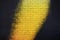# Classification Visualizations with Yellowbrick

## How to visualize classification model performance using Yellowbrick

Whether we are iterating over performance models or presenting to clients, data scientists utilize visualizations regularly. While there are many visualization libraries available to us, Yellowbrick serves as a natural extension to scikit-learn’s modeling process and assists with model interpretation and tuning.

“Visualization gives you answers to questions you didn’t know you had.”

— Ben Schneiderman

This post is to serve as an introduction to Yellowbrick and display a few ways in which it can simplify the process of visualizing the results of various classification models.Photo by Chris Barbalis on Unsplash

I will be using Pandas and NumPy to assist with data frame manipulation and will being using seaborn, ironically, to load in the famous penguin data frame. I will import classifiers and modules as we go for ease of interpretation. The first code block includes standard imports, reading data, and basic data cleaning.

`import pandas as pdimport numpy as npimport seaborn as snsimport warningswarnings.simplefilter(action='ignore', category=FutureWarning)# Loading in data and dropping NaNspenguins = sns.load_dataset('penguins')penguins = penguins.dropna()# Mapping species of penguins to a numerical valuepenguins['species'] = \    penguins['species'].replace({'Adelie': 0,                                  'Chinstrap': 1,                                  'Gentoo': 2})# Mapping sex to a numerical valuepenguins['sex'] = \    np.where(penguins['sex'] == 'Male', 1, 0)# Binarizing 'island'penguins = pd.get_dummies(penguins, drop_first=True)# Viewing a sample of the datapenguins.sample(3)`
`# Creating my input variables, X and target variable, yX = penguins.drop('species', axis=1)y = penguins['species']# Splitting data into training and test sets.  from sklearn.model_selection import train_test_splitX_train, X_test, y_train, y_test = train_test_split(X, y, stratify=y)# Identifying classes. The classes variable will be useful when using Yellowbrick's visualizersclasses = ['Adelie', 'Chinstrap', 'Gentoo']`

## Classification Report

The classification report is a heatmap of your model’s precision, recall and f-1 score on a class basis. The class basis classification report aids in understanding multi-class problems that might be difficult to assess with a global f-1 or accuracy score. Additionally, you may set the argument `support=True` to view the number of actual occurrences in each class in the dataset.

`from yellowbrick.classifier import ClassificationReportfrom sklearn.neighbors import KNeighborsClassifiermodel = KNeighborsClassifier()vizualizer = ClassificationReport(model, classes=classes, support=True)vizualizer.fit(X_train, y_train)vizualizer.score(X_test, y_test)vizualizer.show();`

## Confusion Matrix

Although scikit-learn has a built-in `plot_confusion_matrix` within its metrics library, Yellowbrick's confusion matrix has additional features that may be of benefit. The argument `percent=True` will display the percent of true (or the cell divided by the row total). The `label_encoder` argument will accept a sci-kit learn label encoder or a dictionary.

`from yellowbrick.classifier import ConfusionMatrixcm = ConfusionMatrix(    model, classes=classes,    percent=True    #label_encoder={0: 'Adelie', 1: 'Chinstrap', 2: 'Gentoo'})cm.fit(X_train, y_train)cm.score(X_test, y_test)cm.show();`

## Class Prediction Error

The class prediction error bar graph is one of my favorites. It is a different take on a confusion matrix that is a little less confusing. The graph displays your predictions in a way that is easy to identify errors. Take the graph below for example; we can see from the first column that the model classified a sizable portion of Chinstrap penguins (green) as Adelie. Meanwhile, the Chinstraps were identified perfectly but a lot of them were incorrectly categorized. Using these new insights, one could easily detect the features causing issues and quickly address the problem.

`from yellowbrick.classifier import ClassPredictionErrorvisualizer = ClassPredictionError(    model, classes=classes)visualizer.fit(X_train, y_train)visualizer.score(X_test, y_test)visualizer.show();`

## Feature Importances

Although not restricted to classification problems, the Feature Importances visualizer is a quick way to utilize scikit-learn’s `feature_importances_` attribute. For regression problems where there is no `feature_importances_` attribute, the visualizer will use the model's `coef_` attribute. Yellowbrick's documentation suggests that you set `relative=False` when using a regression model to understand the true magnitude of the coefficient.

`from yellowbrick.model_selection import FeatureImportancesfrom sklearn.ensemble import RandomForestClassifiermodel = RandomForestClassifier()model.fit(X_train, y_train)visualizer = FeatureImportances(model)visualizer.fit(X_train, y_train)visualizer.show();`

## ROC AUC

Below is a Receiver Operating Characteristic/Area Under the Curve plot, or ROC AUC, using Yellowbrick’s `spam` dataset for binary classification. ROC AUC is generally used for binary classification, however, Yellowbrick's ROC AUC does allow for multi-class classification. I chose to feature the spam dataset instead of our beloved penguins because it did a better job displaying the quintessential ROC AUC curve. The graph displays the ROC for each class as well as the micro and macro averages. The micro averages are computed from the sum of all true positives and false positives across all classes and the macro averages are the averages of curves across all classes. See the documentation for more information.

I appreciate the multitude of options within this visualizer. You can toggle between the different curves and have them displayed including their corresponding ROC AUC score in the legend.

`from yellowbrick.classifier import ROCAUCfrom yellowbrick.datasets import load_spamfrom sklearn.linear_model import LogisticRegressionX, y = load_spam()X_train, X_test, y_train, y_test = train_test_split(X, y, stratify=y)model = LogisticRegression(max_iter=10000)model.fit(X_train, y_train)visualizer = ROCAUC(model, classes=['ham', 'spam'])visualizer.fit(X_train, y_train)visualizer.score(X_test, y_test)visualizer.show();`

Thank you for exploring a selection of Yellowbrick’s classification visualizations with me. I have been utilizing these visualizers in my own models and have found them to be convenient and informative. I plan to write another entry devoted to regression visualizations.

Data scientist and classical musician based in Raleigh, NC.

## More from Alex Fioto

Data scientist and classical musician based in Raleigh, NC.